Home

# A SMALL VALUE ESTIMATE FOR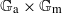$\mathbb {G}_{{\textrm{a}}}\times \mathbb {G}_{{\textrm{m}}}$

## Abstract

A small value estimate is a statement providing necessary conditions for the existence of certain sequences of non-zero polynomials with integer coefficients taking small values at points of an algebraic group. Such statements are desirable for applications to transcendental number theory to analyze the outcome of the construction of an auxiliary function. In this paper, we present a result of this type for the product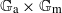$\mathbb {G}_{\mathrm {a}}\times \mathbb {G}_{\mathrm {m}}$ whose underlying group of complex points is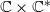$\mathbb {C}\times \mathbb {C}^{*}$ . It shows that if a certain sequence of non-zero polynomials in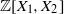$\mathbb {Z}[X_1,X_2]$ takes small values at a point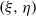$(\xi ,\eta )$ together with their first derivatives with respect to the invariant derivation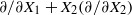$\partial /\partial X_1 + X_2 (\partial /\partial X_2)$ , then both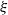$\xi$ and$\eta$ are algebraic over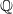$\mathbb {Q}$ . The precise statement involves growth conditions on the degree and norm of these polynomials as well as on the absolute values of their derivatives. It improves on a direct application of Philippon’s criterion for algebraic independence and compares favorably with constructions coming from Dirichlet’s box principle.

## References

Hide All
MathJax
MathJax is a JavaScript display engine for mathematics. For more information see http://www.mathjax.org.

# A SMALL VALUE ESTIMATE FOR$\mathbb {G}_{{\textrm{a}}}\times \mathbb {G}_{{\textrm{m}}}$

## Metrics

### Full text viewsFull text views reflects the number of PDF downloads, PDFs sent to Google Drive, Dropbox and Kindle and HTML full text views.

Total number of HTML views: 0
Total number of PDF views: 0 *Loading metrics...

### Abstract viewsAbstract views reflect the number of visits to the article landing page.

Total abstract views: 0 *Loading metrics...

* Views captured on Cambridge Core between <date>. This data will be updated every 24 hours.

Usage data cannot currently be displayed